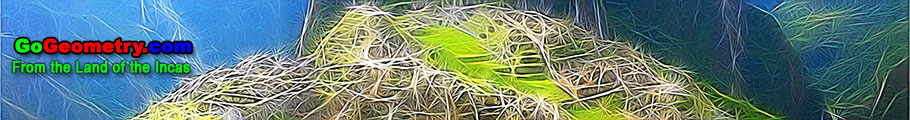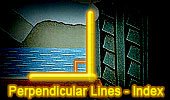Perpendicular Lines, Theorems and Problems - Table of Content  (17)Proposed Problem 230. Triangle, Midpoints, Transversal, Perpendicular lines. Proposed Problem 229. Triangle, Centroid, Transversal, Perpendicular lines, Parallel lines. Proposed Problem 228. Triangle, Midpoints, Exterior line, Perpendiculars lines. Proposed Problem 227. Triangle, Centroid, Exterior line, Perpendicular lines, Parallel lines. Proposed Problem 226. Triangle, Centroid, Perpendicular lines, Parallel lines. Proposed Problem 224. Viviani's theorem, Isosceles triangle, Altitude, Distances, Point on the extension of the base, Perpendicular lines, Parallel lines. Proposed Problem 223. Viviani's theorem, Isosceles triangle, Altitude, Distances, Perpendicular lines, Parallel lines. Proposed Problem 222. Viviani's theorem, Equilateral triangle, Exterior point, Perpendicular lines, Distances. Proposed Problem 221. Viviani's theorem, Equilateral triangle, Interior point, Distances, Parallel lines. Proposed Problem 219. Rhombus, Perpendicular lines, arithmetic mean, Parallel lines. Proposed Problem 218. Right triangle, Altitude and Projections, Perpendicular lines, Parallel lines. Proposed Problem 217. Right triangle, Altitude and Projections, Perpendicular lines, Parallel lines. Proposed Problem 207. Right Triangle, Hypotenuse, Inradius, Exradius relative to the hypotenuse, Perpendicular lines. Problem 206. Area of a Right Triangle, Inradius, and Exradius relative to the hypotenuse, Perpendicular lines. Proposed Problem 205. Right Triangle Area, Exradii relatives to legs or catheti, Perpendicular lines. Go to Page: Previous | 1 | 2 | 3 | 4 | 5 | 6 | 7 | 8 | 9 | 10 | 11 | 12 | 13 | 14 | 15 | 16 | 17 | 18 | 19 | 20 | Next

 Home | Search | Geometry | Post a comment | Email | Post a comment | By Antonio Gutierrez Last updated: Feb 15, 2015